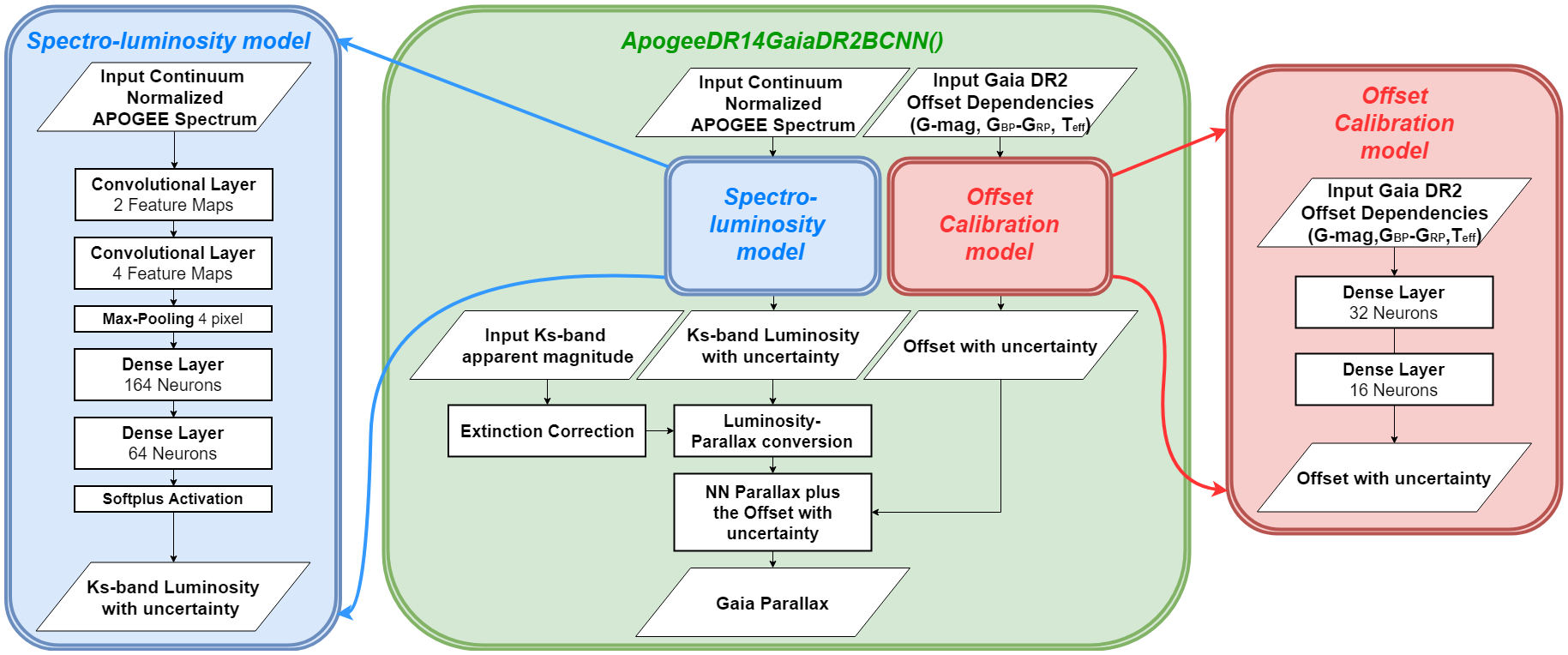# APOGEE Spectra with Bayesian NN and Gaia offset calibration - ApogeeDR14GaiaDR2BCNN

Class for Bayesian convolutional neural network for APOGEE DR14 Gaia DR2

History:

2018-Nov-06 - Written - Henry Leung (University of Toronto)

digraph inheritanceb16186b377 { bgcolor=transparent; dpi=144; rankdir=LR; size="8.0, 12.0"; "ABC" [URL="https://docs.python.org/3/library/abc.html#abc.ABC",dpi=144,fillcolor=white,fontname="Vera Sans, DejaVu Sans, Liberation Sans, Arial, Helvetica, sans",fontsize=10,height=0.25,shape=box,style="setlinewidth(0.5),filled",target="_top",tooltip="Helper class that provides a standard way to create an ABC using"]; "ApogeeDR14GaiaDR2BCNN" [URL="#astroNN.models.apogee_models.ApogeeDR14GaiaDR2BCNN",dpi=144,fillcolor=white,fontname="Vera Sans, DejaVu Sans, Liberation Sans, Arial, Helvetica, sans",fontsize=10,height=0.25,shape=box,style="setlinewidth(0.5),filled",target="_top",tooltip="Class for Bayesian convolutional neural network for APOGEE DR14 Gaia DR2"]; "BayesianCNNBase" -> "ApogeeDR14GaiaDR2BCNN" [arrowsize=0.5,style="setlinewidth(0.5)"]; "BayesianCNNBase" [URL="basic_usage.html#astroNN.models.base_bayesian_cnn.BayesianCNNBase",dpi=144,fillcolor=white,fontname="Vera Sans, DejaVu Sans, Liberation Sans, Arial, Helvetica, sans",fontsize=10,height=0.25,shape=box,style="setlinewidth(0.5),filled",target="_top",tooltip="Top-level class for a Bayesian convolutional neural network"]; "NeuralNetMaster" -> "BayesianCNNBase" [arrowsize=0.5,style="setlinewidth(0.5)"]; "ABC" -> "BayesianCNNBase" [arrowsize=0.5,style="setlinewidth(0.5)"]; "NeuralNetMaster" [URL="basic_usage.html#astroNN.models.base_master_nn.NeuralNetMaster",dpi=144,fillcolor=white,fontname="Vera Sans, DejaVu Sans, Liberation Sans, Arial, Helvetica, sans",fontsize=10,height=0.25,shape=box,style="setlinewidth(0.5),filled",target="_top",tooltip="Top-level class for an astroNN neural network"]; "ABC" -> "NeuralNetMaster" [arrowsize=0.5,style="setlinewidth(0.5)"]; }

ApogeeDR14GaiaDR2BCNN can only be used with Apogee spectra with 7,514 pixels

```from astroNN.models import ApogeeDR14GaiaDR2BCNN

# Load the train data from dataset first, x_train is spectra and y_train will be ASPCAP labels

# And then create an instance of Apogee Censored Bayesian Convolutional Neural Network class

# Set max_epochs to 10 for a quick result. You should train more epochs normally, especially with dropout
apogee_gaia_bcnn.max_epochs = 10
apogee_gaia_bcnn.train(x_train, y_train, x_err, y_err)
```

Here is a list of parameter you can set but you can also not set them to use default

```ApogeeDR14GaiaDR2BCNN.batch_size = 64
# number of neurone for [old_bcnn_1, old_bcnn_2, offset_hidden_1, offset_hidden_2]
ApogeeDR14GaiaDR2BCNN.num_hidden = [162, 64, 32, 16]
```

Note

You can disable astroNN data normalization via `ApogeeDR14GaiaDR2BCNN.input_norm_mode=0` as well as `ApogeeDR14GaiaDR2BCNN.labels_norm_mode=0` and do normalization yourself. But make sure you don’t normalize labels with MAGIC_NUMBER (missing labels).

After the training, you can use apogee_gaia_bcnn in this case and call test method to test the neural network on test data. Or you can load the folder by

```from astroNN.models import load_folder

# Load the test data from dataset, x_test is spectra and y_test will be ASPCAP labels
test_data = ......

# pred contains denormalized result aka. fakemag prediction in this case
# pred_std is a list of uncertainty
# pred_std['total'] is the total uncertainty (standard derivation) which is the sum of all the uncertainty
# pred_std['predictive'] is the predictive uncertainty predicted by bayesian neural net
# pred_std['model'] is the model uncertainty from dropout variational inference
pred, pred_std = apogee_gaia_bcnn.test(test_data)
```
```# Calculate jacobian
jacobian_array = apogee_gaia_bcnn.jacobian(x_test, mean_output=True)
```

## Architecture

The architecture of this neural network is as follow.